# GMAT Math : Calculating the angle for a percentage of a circle

## Example Questions

### Example Question #1 : Circles

How many degrees does the hour hand on a clock move between 3 PM and 7:30 PM?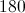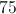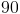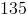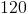Explanation:

An hour hand rotates 360 degrees for every 12 hours, so the hour hand moves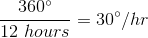.

There are 4.5 hours between 3 PM and 7:30 PM, so the total degree measure is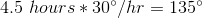.

### Example Question #232 : Problem Solving Questions

If a sector coversof a circle, what is the angle of the sector?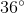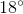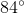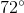Explanation:

One full rotation of a circle is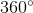, so if a sector coversof a circle, its angle will beof. This gives us: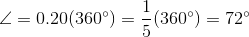### Example Question #1 : Calculating The Angle For A Percentage Of A Circle

A given sector covers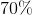of a circle. What is the corresponding angle of the sector?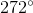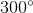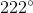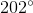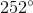Explanation:

A circle comprises, so a sector comprisingof the circle will have an angle that isof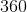.

Therefore: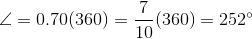### Example Question #2 : Calculating The Angle For A Percentage Of A Circle

A given sector of a circle comprises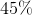of the circle. What is the corresponding angle of the sector?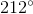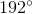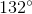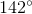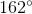Explanation:

A circle comprises, so a sector comprisingof the circle will have an angle that isof.

Therefore: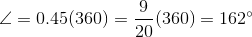### Example Question #3 : Calculating The Angle For A Percentage Of A Circle

The hour hand on a clock moves from 3PM to 6PM. How many degrees does the hour hand move?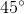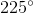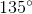Explanation:

The hour hand moves around a circle from 3PM to 6PM. Since there are 12 hours on a clock and the hand is moving through 3 of them, the hand is moving through a sector comprisingof the circle because,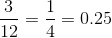.

Since a circle has, the angle of the sector is: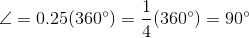### Example Question #4 : Calculating The Angle For A Percentage Of A Circle

The town of Thomasville organized a search party to look for a missing chicken. The party consisted of groups of people choosing a sector and searching outward from the center of town. Find the angle for the sector of searched by each group if each group chose an equal sized sector, and there were 120 groups.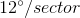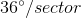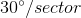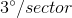Explanation:

The town of Thomasville organized a search party to look for a missing chicken. The party consisted of groups of people choosing a sector and searching outward from the center of town. Find the angle for the sector of searched by each group if each group chose an equal sized sector, and there were 120 groups.

Begin by dissecting the question and figurign out exactly what they are asking and telling you. It's a bit wordy, but what we are looking for is the measure of the central angle for each of the search-sectors

We are told that there are 120 equal sectors.

We also know that a circle is made up ofSo, to find the central angle of each sector, simply do the following calculation: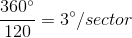Tired of practice problems?

Try live online GMAT prep today.